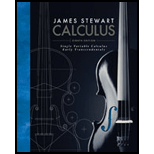Chapter 7.8, Problem 52E### Single Variable Calculus: Early Tr...

8th Edition
James Stewart
ISBN: 9781305270336

#### Solutions

Chapter
Section### Single Variable Calculus: Early Tr...

8th Edition
James Stewart
ISBN: 9781305270336
Textbook Problem

# Use the Comparison Theorem to determine whether the integral is convergent or divergent.52. ∫ 0 ∞ arctan x 2 + e x   d x

To determine

To find: whether the integralfunction is convergent or divergent using Comparison Theorem.

Explanation

Given information:

The integral function is 0arctanx2+exdx.

Calculation:

Show the integral function as follows:

0arctanx2+exdx (1)

Considerf and g are continuous function with f(x)g(x)0 for xa.

Part (a):

If af(x)dx is convergent, then ag(x)dx is convergent.

Part (b):

If ag(x)dx is divergent, then af(x)dx is divergent.

Consider the value of the function g(x)=arctanx2+ex (2)

Consider the value of the function f(x)=2ex (3)

Calculate the value of g(x) within limits [0,] using Equation (2).

Substitute 0 for x in Equation (2).

g(x)=arctanx2+ex=arctan(0)2+e0=0

Substitute for x in Equation (2).

g(x)=arctanx2+ex=arctan()2+e=arctan()=0

Calculate the value of f(x) within limits [0,] using Equation (3).

Substitute 0 for x in Equation (3).

f(x)=2ex=2e0=2

Substitute for x in Equation (3).

f(x)=2ex=2e=0

Compare the value of f(x) and g(x) within limits [0,]

### Still sussing out bartleby?

Check out a sample textbook solution.

See a sample solution

#### The Solution to Your Study Problems

Bartleby provides explanations to thousands of textbook problems written by our experts, many with advanced degrees!

Get Started

## Additional Math Solutions

#### Find more solutions based on key concepts